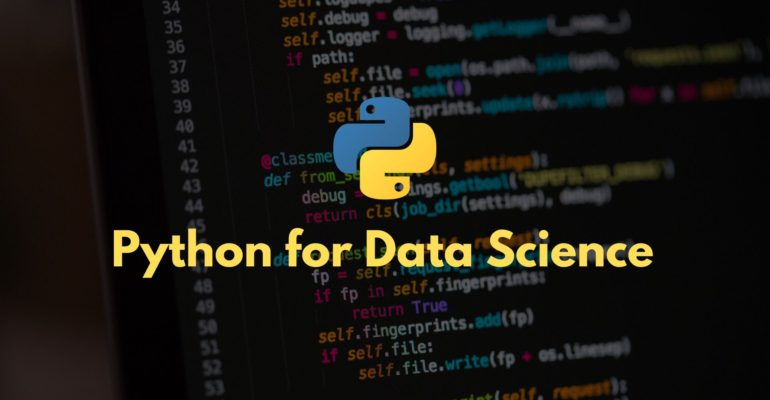Thanks to theidioms.com

## Control Flow Tools in Python# Control Flow Tools in Python

In this chapter, you will learn about various control flow tools used in Python.

Control Flow tools in Python are like the ones used in other programming languages. Control flow statements determine what section of code should run in a program at any time. These statements include conditional statements and loops. Let’s discuss each one of them in detail.

#### 1. If statement

The if statement is a conditional statement responsible for executing programming blocks based on specified conditions.

Example of if, elif and else statement in Python:

```# Program to find whether a given integer is positive, negative or zero

x = int (input( "Please enter an integer: " ))

if x < 0:
print ('Negative Number')
elif x == 0:
print ('Zero')
elif x > 0:
print ('Positive Number')
else:
print ('None') # This is optional here
```
```OUTPUT:
Negative Number```

#### 2. For loop statement

The for loop statement is a control flow tool in python used to iterate over the items of any sequence (a list or a string) in the order that they appear in the sequence. The syntax of for loop in Python is slightly different from what you may have used in other programming languages, such as C or C++.

Example of for statement in Python:

```# Program that iterates over a given list of numbers
# Defining a list of integers
list_of_numbers = [10, 15, 20, 25, 30, 35]

# for statement
for number in list_of_numbers:
print (number)
```
```OUTPUT:
10
15
20
25
30
35```

Using range() function for loops

The range() function generates an arithmetic progression. This function is used for iterating over a sequence of numbers.

Syntax: range(start, stop, step)

where,

• start is the starting value of the range that is inclusive,
• stop is the ending value of the range that is exclusive, and
• step is the value of step used in the progression (optional).

Example of range() function in Python:

```# For statement with range() function

for x in range(10, 40, 5):  # Starting number is with 10 included, stop value is 39, with a step of 5
print (x)```
```OUTPUT:
10
15
20
25
30
35```

#### 3. While loop statement

The While statement is an iterator used to execute a block of statements until the specified condition is matched. It is one of the mostly used control flow tools in Python.

Example of while statement in Python:

```# Declaring a variable x
x = 10

while x < 35:  # run a loop while x is less than 35
x = x + 5
print(x)```
```OUTPUT:
15
20
25
30
35```

#### 4. Break and Continue statement

The break and continue statements alter the flow of a normal loop. The break statement breaks out of the innermost enclosing for or while loop, whereas, the continue statement continues with the next iteration of the loop. In other words, the continue statement escapes the block of the statement below it and starts with the next iteration of the loop.

Example of break and continue statement in Python:

```# Defining a list of elements
actions = ["wake", "wash", "eat", "work", "play", "sleep"]

for action in actions:
if action == "sleep":
break            # if the action is sleep, this would terminate the loop completely
elif action == "play":
continue       # if the action is play, this would not terminate the loop but continues to next iteration
else:
print (action)
```
```OUTPUT:
wake
wash
eat
work```

#### 5. Pass statement

The pass statement is one of those control flow tools in python that does nothing. It can be used when a statement is required syntactically, but the program requires no action.

Example of pass statement in Python:

```# Program that shows the use of a pass function
x = 5
if x == 5:
pass    # this does nothing```
`NO OUTPUT`

This is what we have for control flow tools in Python. In the next chapter, we will discuss the different types of ‘Errors and Exceptions in Python‘.